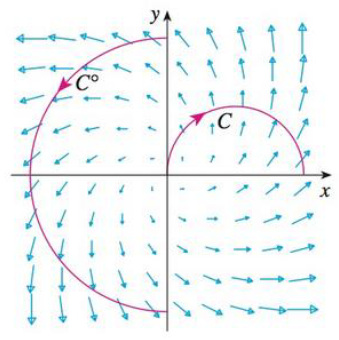Chapter 16.2, Problem 18E

Chapter
Section
Textbook Problem

The figure shows a vector field F and two curves C1 and C2. Are the line integrals of F over C1, and C2 positive, negative, or zero? Explain.To determine

Whether the expression C1Fdr is positive, negative, or zero.

Explanation

Given data:

Refer to Figure in the textbook (i.e., bottom of the question 16.2-18E).

Consider the curve in the quadrants II and III as curve C1 and the curve in the first quadrant as curve C2 .

The curve C1 is the semi-circle which starts at the positive y-axis and ends at the negative y-axis.

Formula used:

Write the expression CFdr for a curve C .

CFdr=CFTds (1)

Here,

T is the unit tangent vector.

Curve C1 :

The field vectors are started on the curve C1 and most of the field vectors in the same direction as the curve C1 .

As the direction of curve C1 is same as the direction of path of the vectors of F , the integration of (FT) is always positive

Still sussing out bartleby?

Check out a sample textbook solution.

See a sample solution

The Solution to Your Study Problems

Bartleby provides explanations to thousands of textbook problems written by our experts, many with advanced degrees!

Get Started

Prove that ddx(secx)=secxtanx

Single Variable Calculus: Early Transcendentals, Volume I

In problems 23-58, perform the indicated operations and simplify. (x3+5x26)(x22)

Mathematical Applications for the Management, Life, and Social Sciences

The area of the region bounded by , , and r = sec θ is:

Study Guide for Stewart's Multivariable Calculus, 8th